## A 5.4 kg bowling ball and a 2.7 gram ping-pong ball collide head-on. If the magnitude of the force by the bowling ball on the ping-pong ball

Question

A 5.4 kg bowling ball and a 2.7 gram ping-pong ball collide head-on. If the magnitude of the force by the bowling ball on the ping-pong ball is 3.5 N, what is the magnitude of the force by the ping-pong ball on the bowling ball

in progress 0
6 months 2021-07-23T05:21:32+00:00 1 Answers 10 views 0

## Answers ( )

The magnitude of force of the ping-pong ball on the bowling ball ,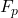= 3.5 N

Explanation:

Given;

mass of the bowling ball,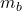= 5.4 kg

mass of the ping-pong ball,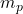= 2.7 g = 0.0027 kg

magnitude of force of the bowling ball,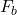= 3.5 N

let the magnitude of force of ping-pong ball =Apply Newton’s third law of motion; action and reaction are equal and opposite.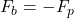The force of the ping-pong ball on the bowling ball is equal to that of the bowling ball on ping-pong ball, but in opposite direction.

Thus, the magnitude of force of the ping-pong ball on the bowling ball ,= 3.5 N